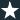Topology ConstraintsYou can define stress (factor of safety) and frequency values as design constraints for a Topology study.

For example, run a Topology study to find the optimal shape of a model with the largest stiffness for a 50% weight reduction and a maximum permissible stress condition. A stress constraint enforces the condition that a model after optimization cannot experience stresses larger than a percentage factor of the material's yield strength.

To specify a stress constraint, in the Goals and Constraints PropertyManager, select Stress/Factor of Safety Constraint. For Type, select either Stress Constraint, or Factor of Safety Constraint.
Stress Constraint
 Specified value Enter the maximum permissible von Mises stress value for the optimized geometry. Specified factor Enter the maximum permissible von Mises stress value as a percentage of the material's yield strength.
Factor of Safety Constraint Enter the minimum acceptable factor of safety for the optimized geometry. The maximum permissible von Mises stress is calculated from the material's yield strength divided by the user-defined factor of safety. The maximum von Mises stress is the default FOS criterion used in this case.

To specify a frequency constraint, in the Goals and Constraints PropertyManager, select Frequency Constraint. Enter a lower or upper, frequency limit, or range of permissible frequencies for the selected mode shapes.

Run a frequency study with the original model (maximum design space), before you run a topology study with frequency constraints, to evaluate the range of permissible natural frequencies.

Select Mode tracking to instruct the optimization solver to track the order of the selected mode shapes derived from the original geometry (throughout the optimization iterations) when enforcing frequency constraints.

When Mode tracking is cleared, the solver tracks the current order of mode shapes as derived for each optimization iteration. For example, it is possible for an optimization goal of a 50% mass reduction and a frequency constraint on the first mode shape. The first mode shape of the original geometry becomes the second or third mode shape of the optimized geometry.

For example, you add a frequency constraint on a distinct mode shape of a plate (the first mode in the original plate geometry). As the model shape changes during iterations, this mode may move down in the frequency list. By selecting Mode tracking, the solver keeps track of the same mode as it moves positions in the list of frequencies, and enforces the constraint on the same mode shape. When you clear Mode tracking, another mode shape replaces the original first mode in the course of iterations. The solver then applies the frequency constraint on this new mode that replaces the old mode.
For a Topology study with a specified frequency constraint only:
• Applied loads or prescribed displacements (including remote loads, translations, and rotations) are not considered in the calculation of the resonant frequencies.
• In the Remote Load/Mass PropertyManager, select Connection Type > Rigid to apply a remote mass. Any remote mass you apply with option Connection Type > Distributed is ignored by the solver.